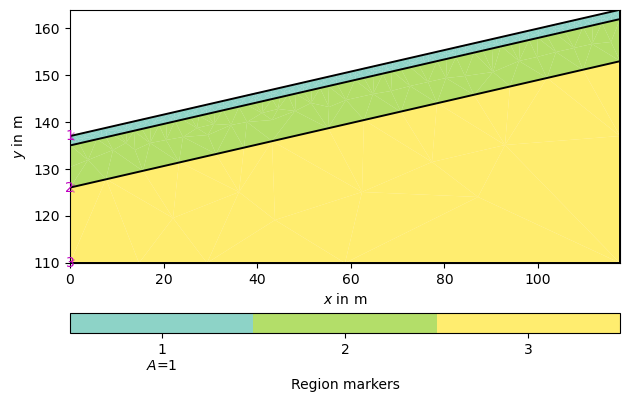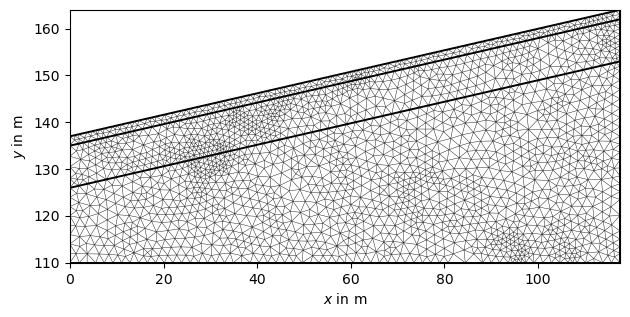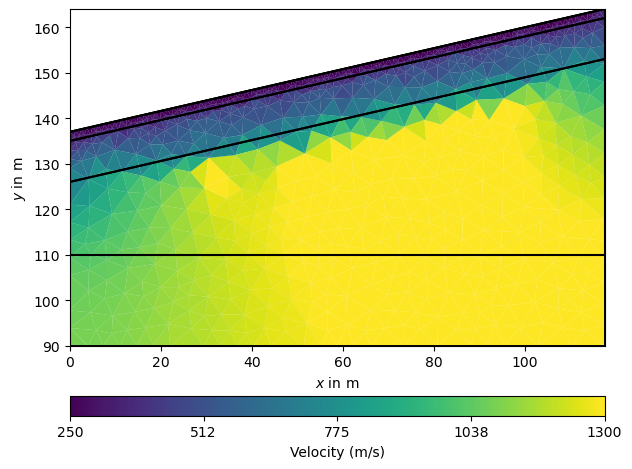# 2D Refraction modeling and inversion#

This example shows how to use the TravelTime manager to generate the response of a three-layered sloping model and to invert the synthetic noisified data.

```import numpy as np

import pygimli as pg
import pygimli.meshtools as mt
from pygimli.physics import TravelTimeManager
```

## Model setup#

We start by creating a three-layered slope (The model is taken from the BSc thesis of Constanze Reinken conducted at the University of Bonn).

```layer1 = mt.createPolygon([[0.0, 137], [117.5, 164], [117.5, 162], [0.0, 135]],
isClosed=True, marker=1, area=1)
layer2 = mt.createPolygon([[0.0, 126], [0.0, 135], [117.5, 162], [117.5, 153]],
isClosed=True, marker=2)
layer3 = mt.createPolygon([[0.0, 110], [0.0, 126], [117.5, 153], [117.5, 110]],
isClosed=True, marker=3)

slope = (164 - 137) / 117.5

geom = layer1 + layer2 + layer3

# If you want no sloped flat earth geometry .. comment out the next 2 lines
# geom = mt.createWorld(start=[0.0, 110], end=[117.5, 137], layers=[137-2, 137-11])
# slope = 0.0

pg.show(geom)

mesh = mt.createMesh(geom, quality=34.3, area=3, smooth=[1, 10])
pg.show(mesh)
```
••```(<matplotlib.axes._subplots.AxesSubplot object at 0x7ff3d56f2c10>, None)
```

Next we define geophone positions and a measurement scheme, which consists of shot and receiver indices.

```numberGeophones = 48
sensors = np.linspace(0., 117.5, numberGeophones)

# Adapt sensor positions to slope
pos = np.array(scheme.sensors())
for x in pos[:, 0]:
i = np.where(pos[:, 0] == x)
new_y = x * slope + 137
pos[i, 1] = new_y

scheme.setSensors(pos)
```

## Synthetic data generation#

Now we initialize the TravelTime manager and asssign P-wave velocities to the layers. To this end, we create a map from cell markers 0 through 3 to velocities (in m/s) and generate a velocity vector. To check whether the model looks correct, we plot it along with the sensor positions.

```mgr = TravelTimeManager()
vp = np.array(mesh.cellMarkers())
vp[vp == 1] = 250
vp[vp == 2] = 500
vp[vp == 3] = 1300

ax, _ = pg.show(mesh, vp, colorBar=True, logScale=False, label='v in m/s')
pg.viewer.mpl.drawSensors(ax, scheme.sensors(), diam=1.0,
facecolor='white', edgecolor='black')
```We use this model to create noisified synthetic data and look at the traveltime data matrix. Note, we force a specific noise seed as we want reproducable results for testing purposes. TODO: show first arrival traveltime curves.

```data = mgr.simulate(slowness=1.0 / vp, scheme=scheme, mesh=mesh,
noiseLevel=0.001, noiseAbs=0.001, seed=1337,
verbose=True)
mgr.showData(data)
``````min/max t: 0.008790995543598544 0.14101993727141843

(<matplotlib.axes._subplots.AxesSubplot object at 0x7ff3d276b250>, None)
```

## Inversion#

Now we invert the synthetic data. We need a new independent mesh without information about the layered structure. This mesh can be created manual or guessd automatic from the data sensor positions (in this example). We tune the maximum cell size in the parametric domain to 15m²

```vest = mgr.invert(data, secNodes=2, paraMaxCellSize=15.0,
maxIter=10, verbose=True)
np.testing.assert_array_less(mgr.inv.inv.chi2(), 1.1)
```
```fop: <pygimli.physics.traveltime.modelling.TravelTimeDijkstraModelling object at 0x7ff3c5a55ea0>
Data transformation: <pygimli.core._pygimli_.RTrans object at 0x7ff3c5a55720>
Model transformation: <pygimli.core._pygimli_.RTransLog object at 0x7ff3c544def0>
min/max (data): 0.0069/0.14
min/max (error): 0.8%/14.67%
min/max (start model): 2.0e-04/0.002
--------------------------------------------------------------------------------
--------------------------------------------------------------------------------
inv.iter 1 ... chi² = 3.81 (dPhi = 99.35%) lam: 20
--------------------------------------------------------------------------------
inv.iter 2 ... chi² = 2.93 (dPhi = 22.14%) lam: 20.0
--------------------------------------------------------------------------------
inv.iter 3 ... chi² = 1.94 (dPhi = 32.21%) lam: 20.0
--------------------------------------------------------------------------------
inv.iter 4 ... chi² = 1.57 (dPhi = 17.47%) lam: 20.0
--------------------------------------------------------------------------------
inv.iter 5 ... chi² = 1.28 (dPhi = 16.57%) lam: 20.0
--------------------------------------------------------------------------------
inv.iter 6 ... chi² = 1.1 (dPhi = 12.24%) lam: 20.0
--------------------------------------------------------------------------------
inv.iter 7 ... chi² = 1.05 (dPhi = 4.97%) lam: 20.0
--------------------------------------------------------------------------------
inv.iter 8 ... chi² = 0.96 (dPhi = 6.93%) lam: 20.0

################################################################################
#                  Abort criterion reached: chi² <= 1 (0.96)                   #
################################################################################
```

The manager also holds the method showResult that is used to plot the result. Note that only covered cells are shown by default. For comparison we plot the geometry on top.

```ax, _ = mgr.showResult(cMin=min(vp), cMax=max(vp), logScale=False)
pg.show(geom, ax=ax, fillRegion=False, regionMarker=False)
``````(<matplotlib.axes._subplots.AxesSubplot object at 0x7ff39f6bfb20>, None)
```

Note that internally the following is called

```ax, _ = pg.show(ra.mesh, vest, label="Velocity [m/s]", **kwargs)
```

Another useful method is to show the model along with its response on the data.

```mgr.showResultAndFit(cMin=min(vp), cMax=max(vp))
``````<Figure size 1100x600 with 6 Axes>
```

Note

Takeaway message

A default data inversion with checking of the data consists of only few lines. Check out Field data inversion (“Koenigsee”).

Total running time of the script: ( 0 minutes 19.530 seconds)

Gallery generated by Sphinx-Gallery